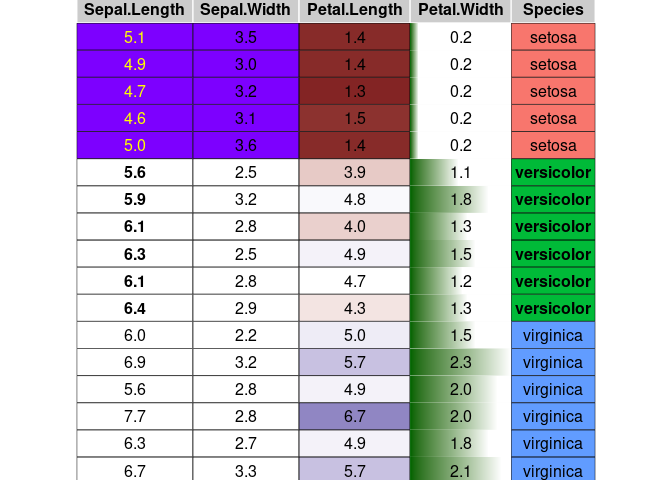Sergio Oller 2023-10-08

condformat renders a data frame in which cells in columns are formatted according to several rules or criteria.

## Browse source code

Checkout the code and browse it at https://github.com/zeehio/condformat.

## How to install condformat:

### Dependencies

If you want to use the PDF output you will need the xcolor LaTeX package. Either use the full texlive distribution, or install latex-xcolor on Debian and derivatives.

### Package installation

• From CRAN:

install.packages("condformat")
• To install the latest development version:

remotes::install_github("zeehio/condformat")

## Example

data(iris)
library(condformat)
condformat(iris[c(1:5,70:75, 120:125),]) %>%
rule_fill_discrete(Species) %>%
rule_fill_discrete(c(Sepal.Width, Sepal.Length),
expression = Sepal.Width > Sepal.Length - 2.25,
colours = c("TRUE" = "#7D00FF")) %>%
rule_text_bold(c(Sepal.Length, Species), Species == "versicolor") %>%
rule_text_color(Sepal.Length,
expression = ifelse(Species == "setosa", "yellow", "")) %>%
rule_fill_bar(Petal.Width, limits = c(0, NA)) %>%
theme_grob(rows = NULL) %>%
condformat2grob()This example covers most of the condformat rules.

## Rules and output engine support

Rule HTML LaTeX gtable Excel
rule_fill_discrete X X X X
rule_fill_gradient X X X X
rule_fill_gradient2 X X X X
rule_text_color X X X X
rule_text_bold X X X X
rule_fill_bar X X
rule_css X